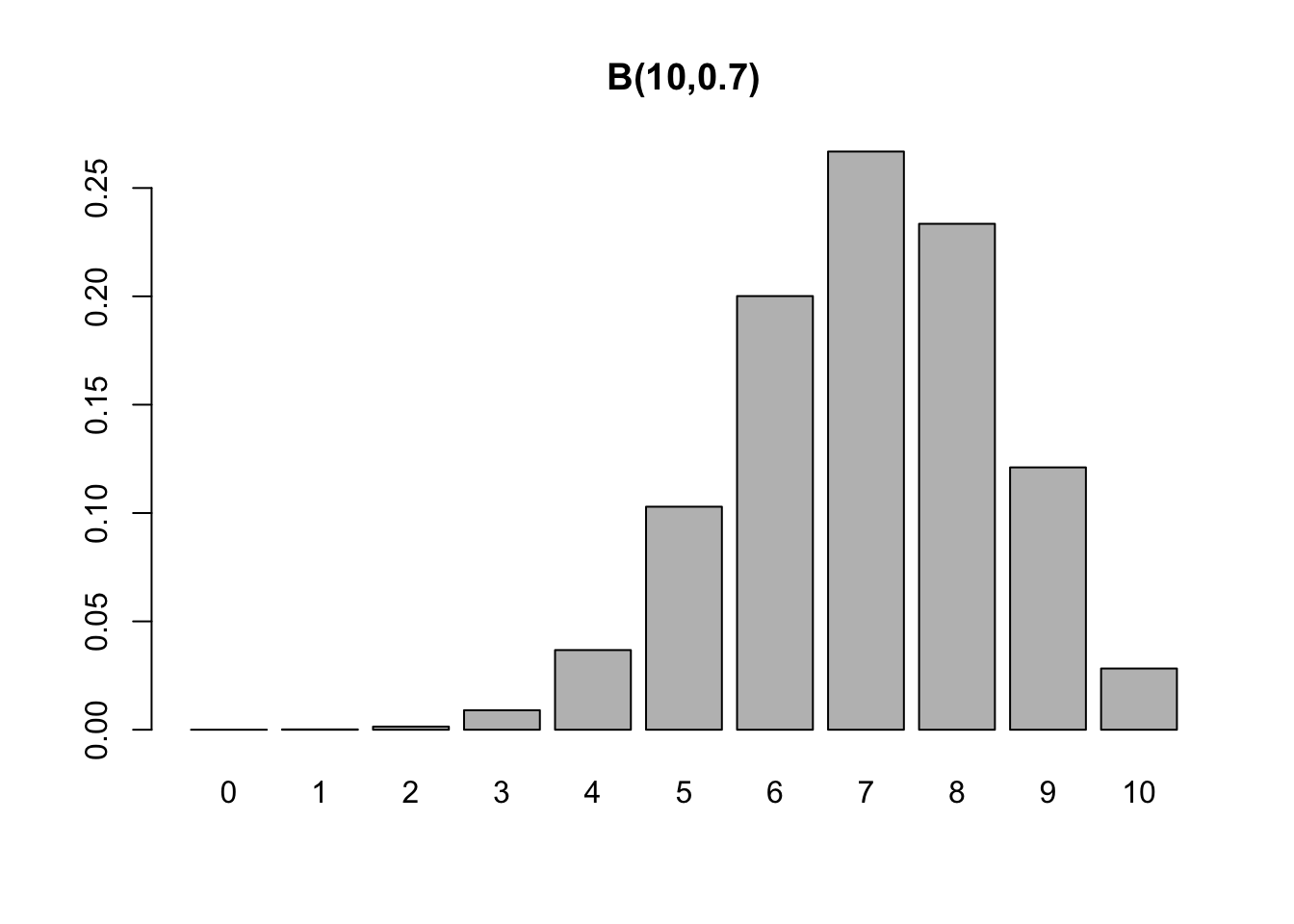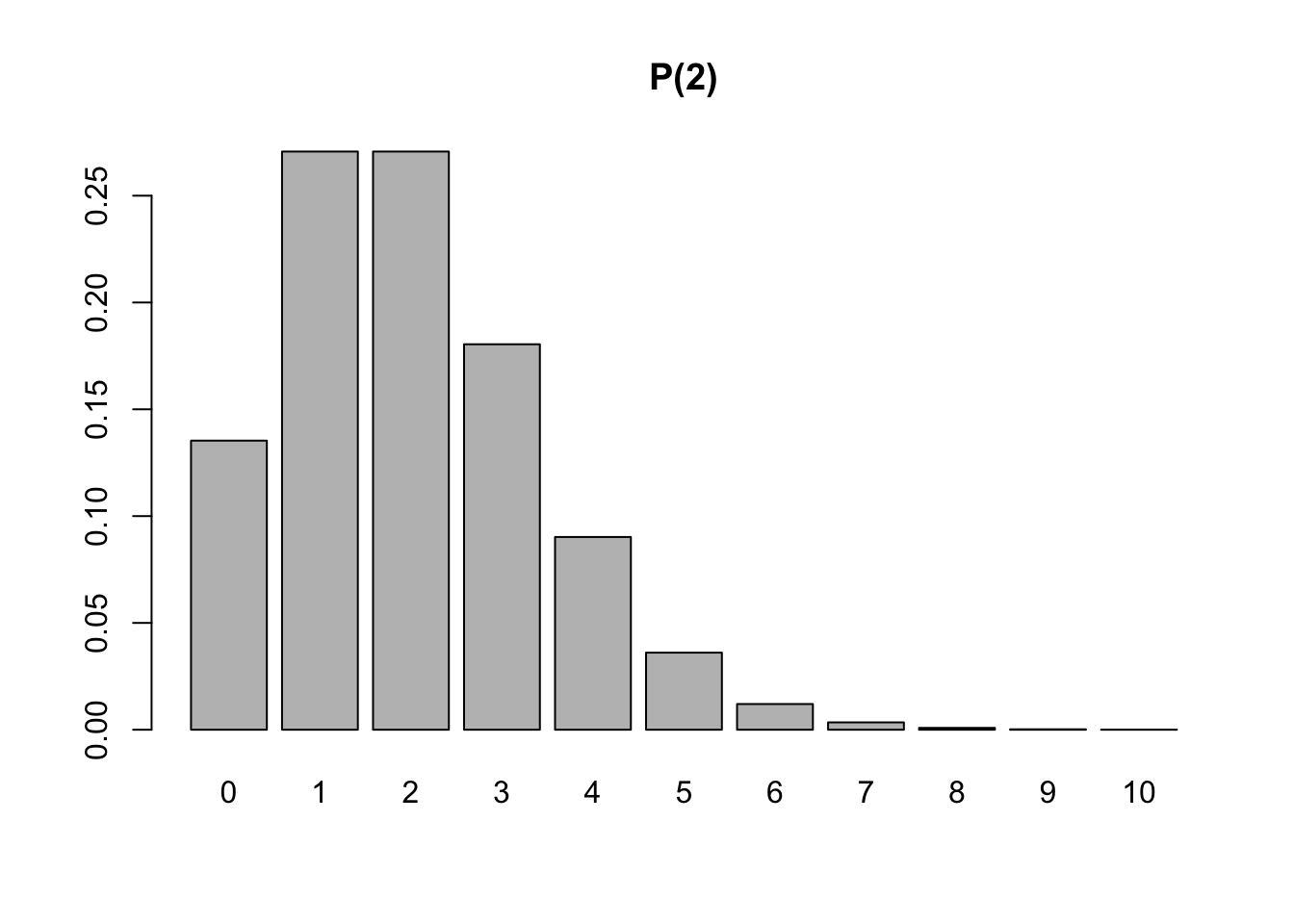## 3.7 Special Discrete Distributions

For more details it is recommended . McLaughlin (2016) brings a compendium of probability distributions.

### 3.7.1Discrete uniform$$\cdot \; \mathcal{DU}(a,b)$$

p(x|a,b) = \tag{3.48} \end{equation}

where $$a$$ and $$b$$ are integers such that $$b \ge a$$.

### 3.7.2Binomial$$\cdot \; \mathcal{B}(n,p)$$

Consider a single toss of a coin that results in heads ($$H$$) or tails ($$T$$). Let $$Pr(\{ H \})=p$$ and $$Pr(\{ T \})=1-p$$. This is a Bernoulli trial. Now suppose $$n$$ independent flips of the same coin, and let $$X$$ be the number of heads resulting in the $$n$$ independent tosses. $$X$$ is a random variable (with probability) binomial distribution of parameters $$n$$ and $$p$$, denoted by $$X \sim \mathcal{B}(n,p)$$. The probability mass function is

$\begin{equation} p(x|n,p) = Pr(X=x) = {n \choose x}p^{x}(1-p)^{n-x} \tag{3.49} \end{equation}$

where $$n \in \mathbb{N}, p \in \left[ 0,1 \right]$$, $$x \in \left\lbrace 0, \ldots, n \right\rbrace$$ and $${n \choose x}$$ is defined by Eq. (3.19).

The expected value and variance are given by

$\begin{equation} E(X)=np \tag{3.50} \end{equation}$

$\begin{equation} V(X)=np(1-p) \tag{3.51} \end{equation}$

Example 3.26 (Binomial) Suppose $$n=10$$ flips of a coin with $$p=0.7$$. Thus, $X \sim \mathcal{B}(10,0.7),$ $p(x) = Pr(X=x) = {10 \choose x} 0.7^{x} 0.3^{10-x},$ $E(X)=10 \times 0.7 = 7,$ $V(X)=10 \times 0.7 \times 0.3 = 2.1.$ %$D(X) = \sqrt{2.1} \approx 1.449138.$

barplot(dbinom(0:10, 10, 0.7), main = 'B(10,0.7)', names.arg = 0:10)### 3.7.3Negative Binomial$$\cdot \; \mathcal{BN}(k,p)$$

Consider again the flip of a coin that results in heads ($$H$$, success) or tails ($$T$$, failure) where $$Pr(\{ H \})=p$$ and $$Pr(\{ T \}) =1-p$$. Let $$X$$ be the ‘number of failures until the $$s$$-th success’. $$X$$ is a random variable with negative binomial distribution of parameters $$s$$ and $$p$$, denoted by $$X \sim \mathcal{BN}(s,p)$$, defined by

$\begin{equation} p(x|k,p) = Pr(X=x) = {x+s-1 \choose x}p^{s}(1-p)^{x} \tag{3.52} \end{equation}$

where $k \in \{1,2,\ldots\}, 0 \le p \le 1, x \in \{0,1,\ldots\}$ and

$\begin{equation} {x+k-1 \choose x} = C_{x}^{x+k-1} = \frac{{(x+k-1)!}}{{x!(k-1)!}} \tag{3.53} \end{equation}$

The expected value and variance are given by

$\begin{equation} E(X)=\frac{pk}{1-p} \tag{3.54} \end{equation}$

$\begin{equation} V(X)=\frac{pk}{(1-p)^2} \tag{3.55} \end{equation}$

Example 3.27 (Negative binomial) A coin with $$p=0.6$$ is tossed until $$k=4$$ heads are obtained. $X \sim \mathcal{BN}(4,0.6),$ $p(x) = Pr(X=x) = {x+3 \choose x} 0.6^{4} 0.4^{x},$ $E(X) = \frac{0.6 \times 4}{1-0.6} = 6$ $V(X)= \frac{0.6 \times 4}{(1-0.6)^2} = 15$ $D(X) = \sqrt{15} \approx 3.873.$

barplot(dnbinom(0:20, 4, 0.6), main = 'BN(4,0.6)', names.arg = 0:20)The functions dnbinom and its corresponding functions (pbinom, qbinom and rbinom) model the variable $$X$$ according to Eq. (3.52). For alternative formulations see this Wikipedia article.

bn <- function(x,k,p){
choose(x+k-1,x) * p^k * (1-p)^x
}
all.equal(bn(0:20, 4, 0.6), dnbinom(0:20, 4, 0.6))
##  TRUE

### 3.7.4Poisson$$\cdot \; \mathcal{P}(\lambda)$$

Siméon Denis Poisson approached the distribution that bears his name considering the limit of a sequence of binomial distributions according to Equation (3.49), in which $$n$$ tends to infinity and $$p$$ tends to zero while $$np$$ remains finitely equal to $$\lambda$$ . The term ‘Poisson distribution’ (Poisson-Verteilung) was coined by , although Abraham de Moivre derived this distribution more than a century before , , .

Example 3.28 Consider a tollbooth where on average $$\lambda$$ vehicles pass per minute. The discrete r.v. $$X$$: ‘number of vehicles per minute’ has Poisson distribution of parameter $$\lambda$$, denoted by $$X \sim \mathcal{P}(\lambda)$$, where $$x \in \left \lbrace 0, 1, 2, \ldots \right\rbrace$$ and $$\lambda > 0$$.

The probability mass function is given by

$\begin{equation} p(x|\lambda) = Pr(X=x) = \frac{{e^{ - \lambda } \lambda ^x }}{{x!}} \tag{3.56} \end{equation}$

where the Euler’s number16 has an approximate value $$e \approx 2.71828\;18284\;59045\;23536$$. The expected value and variance are given by
$\begin{equation} E(X)=\lambda \tag{3.57} \end{equation}$

$\begin{equation} V(X)=\lambda \tag{3.58} \end{equation}$

Example 3.29 (Poisson) Consider a tollbooth where an average of $$\lambda = 2$$ vehicles pass per minute. Thus, $X \sim \mathcal{P}(2),$ $p(x) = Pr(X=x) = \frac{{e^{- 2} 2^{x} }}{{x!}},$ $E(X)=2,$ $V(X)=2.$ $D(X) = \sqrt{2} \approx 1.4142.$

barplot(dpois(0:10, 2), main = 'P(2)', names.arg = 0:10)Exercise 3.16 Consider the Poisson distribution and the exponential function defined by the series $$\sum_{x=0}^{\infty} \frac{\lambda^x}{x!} = e^\lambda$$ . a. Show that the function described by Eq. (3.56) is a probability (mass) function. b. Show that $$E(X)$$ is given by Eq. (3.57). c. Show that $$V(X)$$ is given by Eq. (3.58).

Solution: Chapter 8 $$\\$$

### 3.7.5Hypergeometric$$\cdot \; \mathcal{H}(N,R,n)$$

Suppose an urn with $$N$$ balls of which $$R$$ are marked with a $$\times$$, from which a sample of $$n$$ balls is taken. Let $$X$$ be the number of marbles marked with $$\times$$ of the $$n$$ drawn. $$X$$ has hypergeometric distribution, denoted by $X \sim \mathcal{H}(N,R,n)$ where $$x \in \left\lbrace 0, 1, \ldots, n \right\rbrace$$, $$N \in \{1,2,\ldots\}$$, $$R \in \{1,2,\ldots,N\}$$, $$n \in \{1,2,\ldots ,N\}$$}. Its probability mass function is defined by

$\begin{equation} p(x|N,R,n) = Pr(X=x) = \dfrac{{R \choose x}{N-R \choose n-x}}{{N \choose n}} \tag{3.59} \end{equation}$

The expected value and variance are given by
$\begin{equation} E(X) = n \frac{R}{N} \tag{3.60} \end{equation}$

$\begin{equation} V(X) = n \frac{R}{N} \frac{N-R}{N} \frac{N-n}{N-1} \tag{3.61} \end{equation}$

Example 3.30 (Hypergeometric) Suppose an urn with $$N=15$$ balls, $$R=10$$ marked with a $$\times$$ from which a sample of $$n=7$$ balls is taken.

barplot(dhyper(0:7, 10, 5, 7), main = 'H(15,10,7)', names.arg = 0:7)### 3.7.6 Bernoulli-Poisson $$\cdot \; \mathcal{BP}(p,\lambda_1,\lambda_2)$$

A random variable $$X$$ has a Bernoulli-Poisson distribution parameterized by $$p$$, $$\lambda_1$$ and $$\lambda_2$$ if it assumes a Poisson distribution of rate $$\lambda_1$$ with probability $$p$$ and a Poisson distribution of rate $$\ lambda_2$$ with probability $$1-p$$. Symbolically $$X \sim \mathcal{BP}(p,\lambda_1,\lambda_2)$$, $$x \in \{ 0,1,\ldots \}$$, $$p \in \left[ 0,1 \right]$$, $$\lambda_1,\lambda_2 > 0$$, i.e.,

$\begin{equation} X \sim \left\{ \begin{array}{l} \mathcal{P}(\lambda_1) \;\; \text{with probability} \;\; p \\ \mathcal{P}(\lambda_2) \;\; \text{with probability} \;\; 1-p \\ \end{array} \right. \tag{3.62} \end{equation}$

Its probability mass function is

$\begin{equation} p(x|\lambda_1,\lambda_2) =\frac{e^{-\lambda_1} \lambda_{1}^{x}}{x!} p + \frac{e^{-\lambda_2} \lambda_{2}^{x}}{x!} (1-p) \tag{3.63} \end{equation}$

The expected value and variance are given by

$\begin{equation} E(X) = (\lambda_1 - \lambda_2)p + \lambda_2 \tag{3.64} \end{equation}$

$\begin{equation} V(X) = -(\lambda_1 - \lambda_2)^2 p^2 + \left[ (\lambda_1 - \lambda_2)^2 + \lambda_1 - \lambda_2 \right] p + \lambda_2 \tag{3.65} \end{equation}$

Exercise 3.17 Consider the Bernoulli-Poisson distribution defined by Eq. (3.63). a. Show that the function described by Eq. (3.63) is a probability mass function. b. Show that $$E(X)$$ is given by Eq. (3.64). Hint: $$\sum_{x=0}^{\infty} \frac{\lambda^x}{x!} = e^\lambda$$ c. Show that $$V(X)$$ is given by Eq. (3.65).

Solution: Chapter 8 $$\\$$

### References

Boros, George, and Victor Moll. 2004. Irresistible Integrals: Symbolics, Analysis and Experiments in the Evaluation of Integrals. Cambridge University Press.
Bortkewitsch, L von. 1898. “Das Gesetz Der Kleinen Zahlen.” https://ia803406.us.archive.org/13/items/dasgesetzderklei00bortrich/.
De Moivre, Abraham. 1718. The Doctrine of Chances: Or, a Method of Calculating the Probabilities of Events in Play. 1st ed. London: printe for A. Millar, in the Strand. https://archive.org/details/b30412390.
———. 1756. The Doctrine of Chances: Or, a Method of Calculating the Probabilities of Events in Play. 3rd ed. London: printe for A. Millar, in the Strand. https://archive.org/details/doctrineofchance00moiv.
Johnson, Norman L, Adrienne W Kemp, and Samuel Kotz. 2005. Univariate Discrete Distributions. John Wiley & Sons.
Poisson, Siméon-Denis. 1837. Recherches Sur La Probabilité Des Jugements En Matière Criminelle Et En Matière Civile: Précédées Des règles générales Du Calcul Des Probabilités. Bachelier. https://archive.org/details/bub_gb__8FAjzHfzHgC/mode/2up.
Stigler, Stephen M. 1982. “Poisson on the Poisson Distribution.” Statistics & Probability Letters 1 (1): 33–35. http://www.medicine.mcgill.ca/epidemiology/hanley/statbook/StiglerPoisson.pdf.

1. In literature it can also be known as Napier number, Napier/Neperian constant, among other forms. Not to be confused with the constant of Euler–Mascheroni $$\gamma \approx 0.57721\;56649\;01532\;86061$$.↩︎